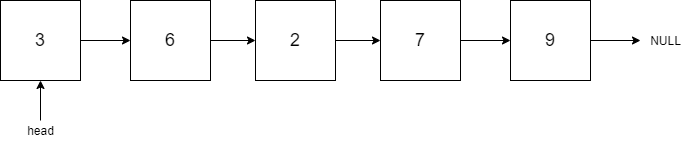New update is available. Click here to update.

# Zero Consecutive Sum Nodes

Last Updated: 19 Mar, 2021
Difficulty: Moderate

## PROBLEM STATEMENT

#### Tanya is preparing for her coding interviews. Recently she discovered about a Singly linked list. A singly linked list is a linear data structure in which we can traverse only in one direction, i.e., from Head to Tail. It consists of several nodes where each node contains some data and a reference to the next node.#### Input Format:

``````The first line of the input contains an integer ‘T’ representing the number of test cases.

The first line of each test case contains space-separated integers denoting the values of nodes of the Linked List. The Linked List is terminated with -1. Hence, -1 is never a node value of the Linked List.
``````

#### Output Format:

``````For each test case, return the head of the linked-list denoting the values of nodes of the singly linked list after removing all consecutive sequences that sum to 0.
``````

#### Note:

``````You do not need to print anything, it has already been taken care of. Just implement the given function.
``````

#### Constraints:

``````1 <= T <= 5
0 <= N <= 100
-10 ^ 3 <= data <= 10 ^ 3 and data != -1

Where 'N' denotes the number of nodes in the given Linked List.

Time Limit: 1 sec
``````